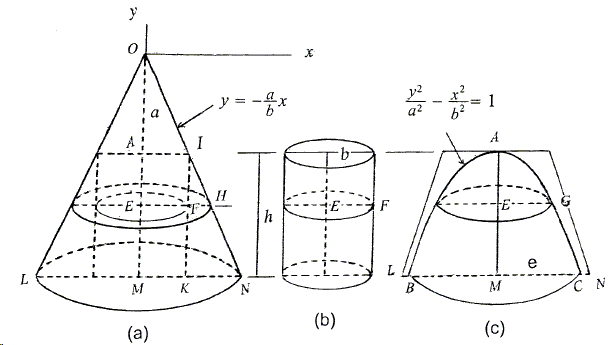A Generalized Cavalieri-Zu Principle
Sidney Kung

# Volume of a Hyperboloid of Two Sheets

A hyperboloid of two sheets is the surface obtained by revolving a hyperbola around its major axis. We are two find the volume as the result of revolving the portion $AC$ of the hyperbola $\displaystyle \frac{x^2}{a^2}-\frac{y^2}{b^2}=1$ and the perpendicular $CM$ around the $y$-axis.In part (a) of the diagram, $OA=a$, $OL$ and $ON$ are asymptotes, $AI=b$. Let $IJ\perp LN$, and let a plane parallel to the base cut the solid, intersecting $OM$, $IJ$, $ON$, and $AC$ at $E$, $F$, $H$, and $G$, respectively. Let $OY=y$ and $EG=x$. Then, since $\displaystyle\frac{b}{a}=\frac{EH}{y}$, or $\displaystyle EH=\frac{b}{a}y$, $\displaystyle x^2=\left(\frac{y^2}{a^2}-1\right)b^{2}=EH^{2}-AI^{2}$, or, $EG^{2}=EH^{2}-EF^2$.

Hence, from (**), the volume of the hyperboloid (for the lower sheet) is the volume of the frustum of a cone minus the volume of the cylinder (parts (c) and (b) in the diagram above). Note that point $C$ is on the hyperbola, $OM=a+h$ so, $\displaystyle (a+h)^{2}=\frac{a^2}{b^2}(b^{2}+e^{2})$, or $\displaystyle h=\frac{a}{b}(\sqrt{b^{2}+e^{2}}-b)$. If $h$ is very large, $MN$ is close to $e$. Thus, we get the volume

$\displaystyle V_{HT}=\frac{1}{3}\pi h(b^{2}+be+e^{2})-\pi b^{2}h=\frac{\pi a}{3b}(e^{2}+be-2b)(\sqrt{b^{2}+e^{2}}-b).$